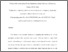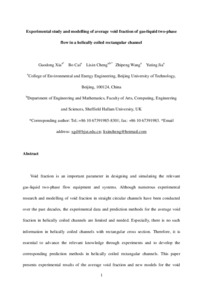# Experimental study and modelling of average void fraction of gas-liquid two-phase flow in a helically coiled rectangular channel

XIA, Guodong, CAI, Bo, CHENG, Lixin, WANG, Zhipeng and JIA, Yuting (2018). Experimental study and modelling of average void fraction of gas-liquid two-phase flow in a helically coiled rectangular channel. Experimental Thermal and Fluid Science, 94, 9-22.Preview
PDF
Cheng-ExperimentalStudyAndModellingOfAvergageVoidFriction(AM).pdf - Accepted Version
Creative Commons Attribution Non-commercial No Derivatives.

Related URLs:

## Abstract

Void fraction is an important parameter in designing and simulating the relevant gas-liquid two-phase flow equipment and systems. Although numerous experimental research and modelling of void fraction in straight circular channels have been conducted over the past decades, the experimental data and prediction methods for the average void fraction in helically coiled channels are limited and needed. Especially, there is no such information in helically coiled channels with rectangular cross section. Therefore, it is essential to advance the relevant knowledge through experiments and to develop the corresponding prediction methods in helically coiled rectangular channels. This paper presents experimental results of the average void fraction and new models for the void fraction in a horizontal helically coiled rectangular channel. First, experiments were conducted with air-water two-phase flow in the horizontal helically coiled rectangular channel at a wide range of test conditions: the liquid superficial velocity ranges from 0.11 to 2 m/s and the gas superficial velocity ranges from 0.18 to 16 m/s. The average void fractions were measured with a quick-closing valve (QCV) method. The measured void fraction ranges from 0.012 to 0.927 which cover four flow regimes including unsteady pulsating, bubbly, intermittent and annular flow observed with a high speed camera. Second, comparisons of the entire measured average void fraction data to 32 void fraction models and correlations were made. It shows a low accuracy of these models and correlations in predicting the experimental data for the void fraction smaller than 0.5 while the drift flux model of Dix (Woldesemayat and Ghajar, 2007) predicts 98.3% of the entire experimental data within ±10% for the void fraction larger than 0.5. Therefore, the Dix model is recommended for the void fraction larger than 0.5. Furthermore, the observed flow regimes in the coiled channels were compared to two mechanistic flow regime maps developed for horizontal straight circular tubes. The flow regime maps do not capture all flow regimes in the present study. Finally, the effects of the limiting affecting parameters on the void fraction models are analyzed according to the physical phenomena and mechanisms. Incorporating the main affecting parameters, new void fraction models have been proposed for the void fractions in the ranges of 0 < α ≤ 0.2 and 0.2 < α ≤ 0.5 respectively according to the slip flow model. Both models predict the experimental data reasonably well. Overall, the new proposed models and the recommended model predict 92.8% of the entire void fraction data within ±30%.

Item Type: Article Air-water two-phase flow; Helically coiled rectangular channel; Flow regime; Void fraction; Experiment; Model Faculty of Science, Technology and Arts > Department of Engineering and Mathematics https://doi.org/10.1016/j.expthermflusci.2018.01.027 9-22 Jill Hazard 06 Feb 2018 11:34 18 Mar 2021 06:38 http://shura.shu.ac.uk/id/eprint/18593View Item